Home
Hostname: page-component-6c8bd87754-sbrr8 Total loading time: 0.181 Render date: 2022-01-20T06:21:52.699Z Has data issue: true Feature Flags: { "shouldUseShareProductTool": true, "shouldUseHypothesis": true, "isUnsiloEnabled": true, "metricsAbstractViews": false, "figures": true, "newCiteModal": false, "newCitedByModal": true, "newEcommerce": true, "newUsageEvents": true }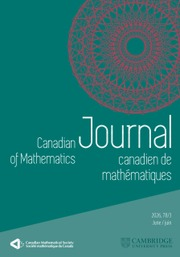Canadian Journal of Mathematics

Special Values of Class Group L-Functions for CM Fields

Published online by Cambridge University Press:  20 November 2018

Abstract

HTML view is not available for this content. However, as you have access to this content, a full PDF is available via the ‘Save PDF’ action button.

Abstract. Let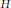$H$ be the Hilbert class field of a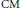$\text{CM}$ number field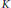$K$ with maximal totally real subfield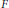$F$ of degree$n$ over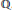$\mathbb{Q}$ . We evaluate the second term in the Taylor expansion at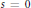$s\,=\,0$ of the Galois-equivariant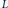$L$ -function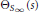${{\Theta }_{{{S}_{\infty }}\,}}\left( s \right)$ associated to the unramified abelian characters of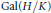$\text{Gal}\left( H/K \right)$ . This is an identity in the group ring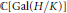$\mathbb{C}\left[ \text{Gal}\left( H/K \right) \right]$ expressing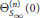$\Theta _{{{S}_{\infty }}}^{(n)}\,\left( 0 \right)$ as essentially a linear combination of logarithms of special values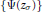$\left\{ \Psi ({{z}_{\sigma }}) \right\}$ , where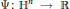$\Psi :\,{{\mathbb{H}}^{n}}\,\to \,\mathbb{R}$ is a Hilbert modular function for a congruence subgroup of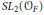$S{{L}_{2}}\left( {{\mathcal{O}}_{F}} \right)$ and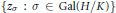$\left\{ {{z}_{\sigma }}\,:\,\sigma \,\in \,\text{Gal}\left( H/K \right) \right\}$ are$\text{CM}$ points on a universal Hilbert modular variety. We apply this result to express the relative class number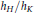${{h}_{H}}/{{h}_{K}}$ as a rational multiple of the determinant of an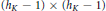$\left( {{h}_{K}}\,-\,1 \right)\,\times \,\left( {{h}_{K}}\,-\,1 \right)$ matrix of logarithms of ratios of special values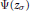$\Psi ({{z}_{\sigma }})$ , thus giving rise to candidates for higher analogs of elliptic units. Finally, we obtain a product formula for$\Psi ({{z}_{\sigma }})$ in terms of exponentials of special values of$L$ -functions.

MSC classification

Type
Research Article
Information
Canadian Journal of Mathematics , 01 February 2010 , pp. 157 - 181

References

[A] T., Asai, On a certain function analogous to log |η(z)|. Nagoya Math J. 40(1970), 193-211.Google Scholar
[B] D., Barsky, Fonctions zeta p-adiques d'une classe de rayon corps de nombres totalement réels. Groupe d'Etude d'Analyse Ultramétrique 16, Secrétariat Math., Paris, 1978.Google Scholar
[BY1] J. H., Bruinier and T., Yang, CM values of Hilbert modular functions. Invent. Math. 163(2006), no. 2, 229-288. doi:10.1007/s00222-005-0459-7Google Scholar
[BY2] J. H., Bruinier and T., Yang, Twisted Borcherds products on Hilbert modular surfaces and their CM values. Amer. J. Math. 129(2007), no. 3, 807-841.Google Scholar
[BF] D., Burns and M., Flach, Motivic L-functions and Galois module structures. Math. Ann. 305(1996), no. 1, 65-102. doi:10.1007/BF01444212Google Scholar
[CN] Cassou-Noguès, P., Valeurs aux entiers négatifs des fonctions zêta et fonctions zêta p-adiques. Invent. Math. 51(1979), no. 1, 29-59. doi:10.1007/BF01389911Google Scholar
[C] C., Chevalley, L'arithmétique dans les algèbres de matrices. Actualités Scientifiques et Industrielles 323, Hermann, Paris, 1936.Google Scholar
[Ch] T., Chinburg, On the Galois structure of algebraic integers and S-units. Invent. Math. 74(1983), no. 3, 321-349. doi:10.1007/BF01394240Google Scholar
[DR] P., Deligne and K., Ribet, Values of abelian L-functions at negative integers over totally real fields. Invent. Math. 59(1980), no. 3, 227-286. doi:10.1007/BF01453237Google Scholar
[GR] I., Gradshteyn, and I., Ryzhik, Table of Integrals, series, and products. Fourth edition, Academic Press, New York-London, 1965.Google Scholar
[K] S., Konno, On Kronecker's limit formula in a totally imaginary quadratic field over a totally real algebraic number field. J. Math. Soc. Japan 17(1965), 411-424.Google Scholar
[Kr] S., Krantz, Function theory of several complex variables. Pure and Applied Mathematics, John Wiley and Sons, Inc., New York, 1982.Google Scholar
[L2] S., Lang, Elliptic Functions. Graduate Texts in Mathematics 112, Springer-Verlag, New York, 1987.Google Scholar
[M] C. J., Moreno, The Chowla-Selberg formula. J. Number Theory 17(1983), no. 2, 226-245. doi:10.1016/0022-314X(83)90022-7Google Scholar
[N] J., Neukirch, Algebraic number theory. Grundlehren der Mathematischen Wissenschaften 322, Springer-Verlag, Berlin, 1999.Google Scholar
[P] C. D., Popescu, Rubin's integral refinement of the abelian Stark conjecture. In: Stark's conjectures: recent work and new directions, Contemp. Math. 358, American Mathematical Society, Providence, RI, 200, pp. 1-35.Google Scholar
[R] K., Rubin, A Stark conjecture ”;over Z“ for abelian L-functions with multiple zeros. Ann. Inst. Fourier (Grenoble) 46(1996), no. 1, 33-62.Google Scholar
[Si] C. L., Siegel, Lectures on advanced analytic number theory. Notes by S. Raghavan. Tata Institute of Fundamental Research Lectures on Mathematics 23, Tata Institute of Fundamental Research, Bombay, 1965.Google Scholar
[St1] H. M., Stark, L-functions at s = 1. I. L-functions for quadratic forms. Advances in Math. 7(1971), 301-343. doi:10.1016/S0001-8708(71)80009-9Google Scholar
[St2] H. M., Stark, L-functions at s = 1. II. Artin L-functions with rational characters. Advances in Math. 17(1975), no. 1, 60-92. doi:10.1016/0001-8708(75)90087-0Google Scholar
[St3] H. M., Stark, L-functions at s = 1. III. Totally real fields and Hilbert's twelfth problem. Advances in Math. 22(1976), no. 1, 64-84. doi:10.1016/0001-8708(76)90138-9Google Scholar
[St4] H. M., Stark, [H. M. Stark] L-functions at s = 1. IV. First derivatives at s = 0. Adv. in Math. 35(1980), no. 3, 197-235. doi:10.1016/0001-8708(80)90049-3Google Scholar
[T] J., Tate, Les conjectures de Stark sur les fonctiones L d'Artin en s = 0. Progress in Mathematics 47, Birkhäuser Boston, Inc., Boston, MA, 1984.Google Scholar
[Y] T., Yang, CM number fields and modular forms. Pure Appl. Math. Q. 1(2005), no. 2, part 1, 305-340.Google ScholarYou have Access

Send article to Kindle

Note you can select to send to either the @free.kindle.com or @kindle.com variations. ‘@free.kindle.com’ emails are free but can only be sent to your device when it is connected to wi-fi. ‘@kindle.com’ emails can be delivered even when you are not connected to wi-fi, but note that service fees apply.

Find out more about the Kindle Personal Document Service.

Special Values of Class Group L-Functions for CM Fields
Available formats
×

Send article to Dropbox

To send this article to your Dropbox account, please select one or more formats and confirm that you agree to abide by our usage policies. If this is the first time you use this feature, you will be asked to authorise Cambridge Core to connect with your <service> account. Find out more about sending content to Dropbox.

Special Values of Class Group L-Functions for CM Fields
Available formats
×

To send this article to your Google Drive account, please select one or more formats and confirm that you agree to abide by our usage policies. If this is the first time you use this feature, you will be asked to authorise Cambridge Core to connect with your <service> account. Find out more about sending content to Google Drive.

Special Values of Class Group L-Functions for CM Fields
Available formats
×
×